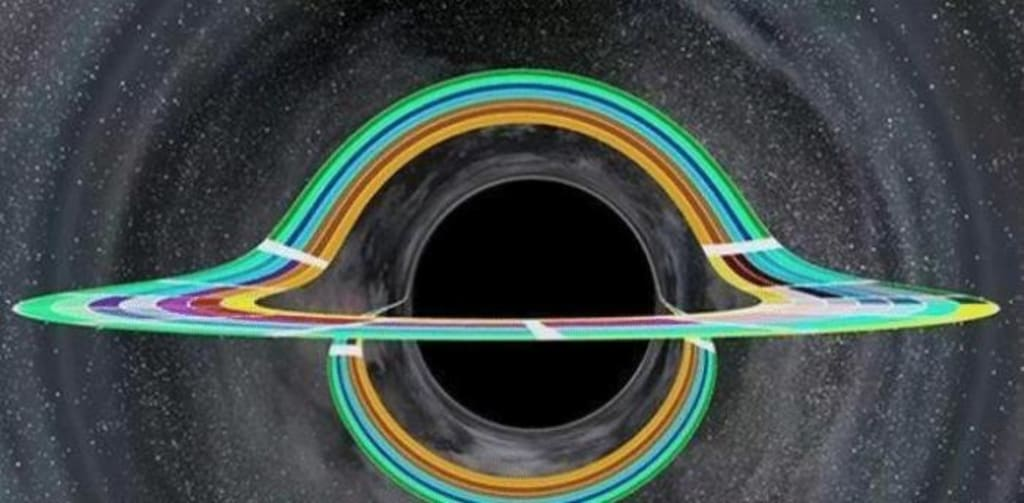# Einstein was right, "it" does exist, the Hubble telescope captured the real picture

## "It does exist, the Hubble telescope took real pictures

By Orr HirshmanPublished 6 months ago 4 min read
LikeThis photo is the most representative picture taken by Hubble, you can see the Einstein ring, In the following, we do a brief introduction to this picture, the first point, this photo is indeed the real Hubble shot, not a computer synthesis PS, such a scene is also real.

We see a glowing circle around the halo, but it is the light from behind the object in the center of the halo. The center of the halo is a very large mass of galactic structures, why would such a phenomenon be formed? This is the result of the gravitational lensing effect. Gravitational lensing is a phenomenon based on general relativity, where light passing through a massive object is bent in time, and the greater the mass, the greater the bending. When a celestial body is massive enough, it gathers the light behind it to the front, creating this phenomenon of magnification or double shadow.

Since this phenomenon is similar to when light passes through a lens, it is called the gravitational lens effect, and light can be bent by this phenomenon, which is very contrary to our first intuition, after all, our common sense perception has always been that we cannot see what is blocked behind by an object with the naked eye alone, but now general relativity tells us that as long as the mass of the object in front is large enough, it will bend the light from the object behind This is one of the special effects of general relativity, light deflection.

In 1919, the British physicist Eddington experimentally verified one of the special effects of the general theory of relativity. It is that light does deflect when it passes through a massive object. This is one of the four major verifications of general relativity, so why would light be bent? With what we know about gravity, what would it take for something like light to be bound by gravity?

Yes, it is indeed difficult to explain with Newton's law of gravity, but general relativity tells us that it is not caused by gravity because gravity does not exist, gravity is only a phenomenon manifested by the bending of spacetime. The essence of light being bent is that light propagates along the geodesic line in the bent space-time, which means the shortest straight line between two points.

In our impression, light travels along a straight line, but the essence is that light travels along the shortest route. In Euclidean geometry, which is what we call the plane, the shortest distance between two points is of course a straight line, but in curved space, which is non-Euclidean geometry, the shortest distance between two points is this side geodesic. So in the perspective of light, the route it travels does not change, it still follows the shortest distance, but space-time is bent. So what we see is the propagation route of light in curved space-time.

This is the latest simulation of the scientists of the black hole dynamic diagram, from this figure can see the perfect Einstein ring, the black hole itself is completely non-luminous, and we now see the middle of the circle of light is the black hole absorption of matter produced by the accretion disk emitted by the light, the upper and lower circle of light around the circle is the gravitational lens formed by the Einstein ring. Because of the powerful gravitational force of the black hole, making extreme distortion of space-time, the light from the accretion disk behind the black hole is bent to the front of the line of sight, forming this circular structure, so no matter from which direction you can see this halo.

Although Einstein predicted the light deflection effect, he overlooked its feasibility as well as its importance in the issue of gravitational lensing. In 1936, he published an article in a scientific journal called "Lensing-like Phenomena of Stars Deflecting Light through Gravitational Fields", but it was calculated by Mandel, a Czech engineer, and because he was not famous, he commissioned Einstein to publish it instead. Einstein did publish it, but he argued that this gravitational lensing phenomenon was not observable because the gravitational fields of stars were not yet strong enough.

In 1937, Zwicky, an astronomer at Caltech, published an article pointing out what Einstein had missed, that the gravitational force of a single star might not be possible, but the gravitational lensing formed by the structure of a galaxy of hundreds of millions of stars could be observed, and Zwicky also pointed out that the mass of such a huge galactic structure could be calculated by the gravitational lensing effect, and that the magnification of the gravitational lensing could also be used to The effect of gravitational lensing can also be used to see faint objects that cannot be observed.

Nowadays, Zwicky's knowledge of gravitational lensing is very profound. The gravitational lensing effect has now become a very important observation tool in cosmology, like those dark matter that we cannot see, we can observe its existence and calculate its mass by the gravitational effect, and for those black holes without accretion disks, we can also use the gravitational lensing effect to determine its existence. But who would have thought that all these are the result of the wisdom of more than 100 years ago?

Science
Like

### Orr Hirshman

How does it work?

There are no comments for this story

Be the first to respond and start the conversation.